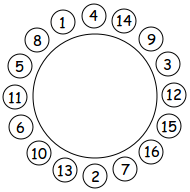CSES - Datatähti Open 2017 - Family reunion
• Time limit: 10.00 s
• Memory limit: 512 MB
There are $n$ people attending Uolevi's family reunion. Soon it is time to move to the dining room where $m$ round tables are reserved for the participants. Each table has places for $n/m$ people, and there are three possible menus (A, B and C).

Each person has a unique identifier between $1 \ldots n$, and they can also see the identifiers of $k$ nearest people to the left and to the right at the table.

It is awkward if two people sitting next to each other choose the same menu. Hence, your task is to invent a strategy that prevents this. Uolevi explains the strategy for all participants beforehand, and everybody follows the same strategy.

Input

The first input line contains three numbers $n$, $m$ and $k$: the number of people and tables, and the seeing distance.

After this, there are $m$ lines, one line for each table. Each line contains $2k+1$ numbers that describe a group of people who sit next to each other at the table.

Output

You should output for each table a single letter A, B or C in a separate line: the menu that the person in the middle chooses.

This task has a special grader: in each test case, your program will be executed several times (at most 50 times). The seating arrangement is fixed for all executions, and the order of the tables is always the same.

Your program will be accepted, if it chooses menus such that no people sitting next to each other will get the same menu.

Example

Consider the following situation ($n=16,m=1,k=7$):Assume that the program will be executed two times, first for person 14 and then for person 4. They are sitting next to each other, so the program must choose different menus for them. For example, the program could choose the menus as follows:

Input:
16 1 7 2 7 16 15 12 3 9 14 4 1 8 5 11 6 10

Output:
B

Input:
16 1 7 7 16 15 12 3 9 14 4 1 8 5 11 6 10 13

Output:
A

This means that person 14 chooses menu B, and person 4 chooses menu A.

• $n = 16$
• $m = 1$
• $k = 7$
• $n=1000$
• $m = 10$
• $k = 7$
• $n=10^9$
• $m = 1000$
• $k = 3$